# KSEEB Solutions for Class 8 Maths Chapter 8 Linear Equations in One Variable Additional Questions

Students can Download Maths Chapter 8 Linear Equations in One Variable Additional Questions and Answers, Notes Pdf, KSEEB Solutions for Class 8 Maths helps you to revise the complete Karnataka State Board Syllabus and score more marks in your examinations.

## Karnataka State Syllabus Class 8 Maths Chapter 8 Linear Equations in One Variable Additional Questions

Question 1.
a. The value of ‘x’ in the equation 5x – 35 = 0 is
A. 2
B. 7
C. 8
D. 11
Solution:
(B) 7

b. If 14 is taken away from one fifth of a number, the result is 20. The equation expressing this statement is
A. $$\left(\frac{x}{5}\right)-14=20$$
B. $$x-\left(\frac{14}{5}\right)=\frac{20}{5}$$
C. $$x-14=\left(\frac{20}{5}\right)$$
D. $$x+\left(\frac{14}{5}\right)=20$$
Solution:
A. $$\left(\frac{x}{5}\right)-14=20$$

c. If five times a number is increased by 8 is 53, the number is
A. 12
B. 9
C. 11
D. 2
Solution:
B. 9

d. The value of x in the equation 5 (x – 2) = 3 (x – 3) is
A. 2
B. $$\frac{1}{2}$$
C. $$\frac{3}{4}$$
D. 0
Solution:
B. $$\frac{1}{2}$$

e. If the sum of two numbers is 84 and their difference is 30, the numbers are
A. – 57 and 27
B. 57 and 27
C. 57 and – 27
D. – 57 and – 27
Solution:
(B) 57 and 27

f. If the area of a rectangle whose length is twice its breadth is 800 m2, then the length and breadth of the rect-angle are
A. 60 m and 20 m
B. 40 m and 20m
C. 80 m and 10 m
D. 100 m and 8m
Solution:
(B) 40 m and 20 m

g. If the sum of three consecutive odd numbers is 249, the numbers are
A. 81, 83, 85
B. 79, 81, 83
C. 103, 105, 10
D. 95, 97, 99
Solution:
(A) 81, 83, 85

h. If $$\frac{(x+0.7 x)}{x}$$ = 0.85 the value of x is
A. 2
B. 1
C. – 1
D. 0
Solution:
B. 1

i. If 2x – (3x – 4) = 3x – 5 then x equals
A. $$\frac{4}{9}$$
B. $$\frac{9}{4}$$
C. $$\frac{3}{2}$$
D. $$\frac{2}{3}$$
Solution:
B. $$\frac{9}{4}$$Question 2.
Solve
(i) $$\frac{(3 x+24)}{(2 x+7)}=2$$
Solution
$$\frac{(3 x+24)}{(2 x+7)}=2$$
3x + 24 = 2( 2x + 7)
3x + 24 = 4x + 14
24 – 14 = 4x – 3x
10 = x
or x = 10

(ii) $$\frac{(1-9 y)}{(11-3 y)}=\frac{5}{8}$$
Solution
$$\frac{(1-9 y)}{(11-3 y)}=\frac{5}{8}$$
8 (1 – 9y) = 5 ( 11 – 3y)
8 – = 55 – 15y
8 – 55 = 72 y – 15 y
– 47 = 57 y
$$\frac{-47}{57}$$ = y
or y = $$\frac{-47}{57}$$

Question 3.
The sum of two numbers is 45 and their ratio is 7 : 8. Find the numbers.
Solution:
Ratio of the number = 7 : 8
Let the numbers be 7x and 8x
sum of the numbers = 45
7x + 8x = 45
15x = 45
x = $$\frac{45}{15}$$
7x = 7 x 3 = 21, 8x = 8 x 3 = 24
∴ The numbers are 21 and 24Question 4.
Shona’s mother is four times as old as shona. After 5 years,her mother will be three times as old as shona (at that time). What are their present age?
Solution:
Let shona’s present age be ‘x’ years.
Her mother’s present age = 4x years
5 years later shona’s age = x + 5 and
shona’s mother’s age = 4x + 5
Given that 4x + 5 = 3 (x + 5)
4x + 5 = 3x + 15
4x – 3x = 15 – 5
x = 10
Shonas present age = x = 10 years
Her mothers present age = 4x = 4 x 10 = 40 years.

Question 5.
The sum of 3 consecutive even numbers is 336. Find them.
Solution:
‘ Let the first even number be ‘x’
The consecutive even numbers are x + 2 and x + 4
Their sum = 336
x + x + 2 + x + 4 = 335
3x + 6 = 336
3x = 336 – 6
3x = 330
x = $$\frac{330}{3}$$ = 110
x = 110, x + 2 = 110 + 2
= 112, x + 4 = 110 + 4 = 114.
∴ The numbers are 11, 112, 114.Question 6.
Two friend’s A and B start a joint business with a capital Rs. 60,000. If As share is twice that of B how much have each invested?
Solution:
Let ‘B’ Invest Rs. x. Then ‘A’s investment is 2x.
Total Investment = 60,000
x + 2x = 60,000
3x = 60,000
x = $$\frac{60000}{3}$$
x = 20,000
‘B’s investment = x = Rs. 20,000
As investment = 2x = 2 x 20,000 = Rs. 40,000

Question 7.
Which is the number when 40 is subtracted gives one third of the original number.
Solution:
Let the number be ‘x’. One third the
$$\frac{1}{3}$$ x number is x = $$\frac{x}{3}$$
Given that x – 40 = $$\frac{x}{3}$$
3 (x – 40) = x
3x – 120 = x
3x – x = 120
2x = 120
x = $$\frac{120}{2}$$
x = 60
∴ The original number is 60

Question 8.
Find the number whose 6th part exceeds its eighth part by 3.
Solution:
Let the number be ‘x’. Its 6th part is $$\frac{x}{6}$$ and 8th part is $$\frac{x}{8}$$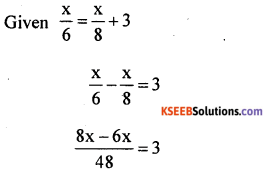x = 72, The number is 72Question 9.
A house and a garden together cost Rs. 8,40,000. The price of the garden is $$\frac{5}{12}$$ times the total cost. Find the price of the house and the garden.
Solution:
The price of the garden is
$$\frac{5}{12}$$ × 8,40,000 . 12
= 5 × 70,000
= Rs. 3,50,000
The cost of the house is 8,40,000 – 3,50,000 = 4,90,000
The cost of the house is Rs. 4,90,000

Question 10.
Two farmers A and B together own a stock of grocery. They agree to divide it by value. Farmer A takes 72 bags while B takes 92 bags and gives Rs. 8,000 to A. What is the cost o each bag?
Solution:
Let the cost of each bag be ‘x’.
A takes 72 bags and Receives Rs. 8000
∴ The value of the stock = 72x + 8000
B takes 92 bags and gives Rs. 8000
The value of the stock = 92x – 8000
∴ 72x + 8000 = 92x – 8000
8000 + 8000 = 92x – 7x
16000 = 20x
∴ x = $$\frac{16000}{20}$$
x = 800
∴ The cost of each bag is Rs. 800Question 11.
A fathers age is four times that of his son. After 5 year s, it will be three times that of his son. How many more years will take if fathers age is to be twice that of his son?
Solution:
Let the sons age be x. Fathers age is 4x .
After 5 years sons age = x + 5 years and fathers age = 4x + 5
Given (4x + 5) = 3 (x + 5)
4x + 5 = 3x + 15 4x – 3x = 15 – 5 x = 10
Sons present age = 10 years
Fathers present age = 4 × x = 4 × 10 = 40 years.
Let in ‘y’ years fathers age will be twice as his sons age.
After ‘y’ years son will be (10 + y) years and father will be (40 + y) years.
40 + y = 2 (10 + y)
40 + y = 20 + 2y
40 – 20 = 2y – y
20 = y
y = 20 ∴ 20 – 5 = 15 years
In 15 years fathers age wi11 be twice his sons age.

Question 12.
Find a number which when multiplied by 7 is as much above 132 as it was origina11y below it.
Solution:
Let the number be x. When its multi, plied by 7 the product is 7x. This number is above 132 by (7x – 12). This is same as it was origina11y below it i.e., (132 – x)
∴ 7x – 132 = 132 – x
7x + x = 132 + 132
8x = 264
x = $$\frac{264}{8}$$ ; x = 33
The number is 33Question 13.
A person buys 25 pens worth Rs. 250, each of equal cost. He wants to keep 5 pens for himself and se11 the remaining to recover his money what should be the price of each pen?
Solution:
Number pens bought = 25
The cost of 25 pens = Rs. 250
∴ The cost of each pen
$$\frac{250}{25}$$ = Rs. 10
He keeps 5 pens with him and se11s the remaining (25 – 5) 20 pens to recover the same amount. Let the S.R of each pen be x.
20x = 250
x = $$\frac{250}{20}$$ = Rs. 12.5
The price of each pen should be Rs. 12.50

Question 14.
The sum of the digits of a two digit number is 12. If the new number formed by reversing the digits is greater than the original number by 18. Find the original number. Check your solution.
Solution:
Let the digit in the units place be ‘x’ and digit in the ten’s place be ‘y
Sum of the digits = 12
x + y = 12
y = 12 – x
∴ The original number is 10 y + x
The number got by reversing the digits is 10 x + y
Given that 10 x + y = 10 y + x + 18
10x + (12 – x ) = 10 (12 – x) + x 18
10x + 12 – x = 120 – 10 x + x + 18
9x + 12 = 138 – 9x
9x + 9x = 138 – 12
18x = 126
x = $$\frac{126}{18}$$ = 7
y = 12 – x
= 12 – y = 5
∴ The original number is (10 x 5) +7 = 50 + 7 = 57

Verification :
Original number = 57
The new number = 75
75 – 57 = 18Question 15.
The distance between two stations is 340 km. Two trains start simaltaneously from these stations on para11el tracks and cross each other. The speed of one of them is greater than that of the other by 5km / hr. If the distance between two trains after 2 hrs of their start is 30 km find the speed of each train.
Solution:
Let the speed of one train be x km /hr. The speed of the other train is (x + 5) km / hr
Distance covered by the trains in 2 hrs are 2x and 2 (x + 5) respectively. After crossing each other the distance between the trains is 30 km. Therefore the total distance covered = 340 + 30 = 370 km
2x + 2 (x + 5) = 370
2x + 2x + 10 = 370
4x + 10 = 370
4x = 370 – 10
4x = 360
x = $$\frac{360}{4}$$ = 90
x = 90 km / hr
The speeds of the trains are 90 km/hr and 90 + 5 = 95 km/ hr.

Question 16.
A steamer goes down stream and coveres the distance between two ports in 4 hrs while it coveres the same distance upstream in 5 hrs. If the speed of the steamer up stream is 2km / hr find the speed of the steamer in sti11 water.
Solution
Speed upstream = 2km / hr
Time taken up stream = 5 hr
∴ Distance between two ports
= 2 x 5 = 10 km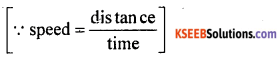Time taken down stream = 4 hrs Distance between two ports = 10 km
speed down streamLet the speed of the steamer in sti11 water be ‘x’ km / hr and speed of the stream be y km / hr.x = 2.25 km / hr
∴ The spped of the steamer in sti11 water is 2.25 km / hr.Question 17.
The numerator of rational number is less than its denaminator by 3. If the numerator becomes 3 times and denominator is increased by 20 the new number becomes $$\frac{1}{8}$$. Find the original number.
Solution:
Let the denominator be ‘x. The numera-tor is x – 3. The original fraction is $$\frac{x-3}{x}$$
If the numerator becomes 3 times it is 3 (x – 3) and denominator is increased by 20 it is x + 20 new number is – $$\frac{1}{8}$$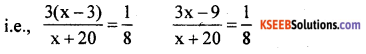8(3x – 9) = x + 20
24x – 72 = x + 20
24x – x = 20 + 72
23x = 92
x = $$\frac{92}{23}$$
x = 4
∴ original fraction = $$\frac{x-3}{x}=\frac{4-3}{4}=\frac{1}{4}$$

Question 18.
The digit at the tens place of a two digit number is three times the digit at the units place. If the sum of this number and the number formed by reversing the digits is 88 find the number.
Solution:
Let the digit in the units place be x and tens place be y.
Given y = 3x ∴ The original number is 10 y + x
The number formed by reversing the digits is 10 x + y.
Sum of the numbers = 88
10 y + x + 10 x + y = 88
11y + 11x = 88
but y = 3x
∴ 11 (3x) + 11x = 88
33x + 11x = 88
44x = 88
x = $$\frac{88}{44}$$
x = 2
y = 3x = 3 x 2 = 6
The original number is 10 y + x = 10 x 6 + 2 = 62Question 19.
The altitude of a triangle is five thirds the length of its corresponding base. If the altitude is increased by 4cm and the base decreased by 2 cm the area of the triangle would remain the same. Find the base and altitude of the triangle.
Solution:
Let the base of the triangle be ‘x’ cm
The altitude is $$\frac{5x}{3}$$ cm
Area of the traingle = $$\frac{1}{2}$$ x base x height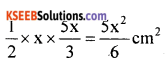If the height is increased by 4cm it is $$\left(\frac{5 x^{2}}{3}+4\right)$$ and the base is decreased by 2 cm it is (x – 2) the area remains the same.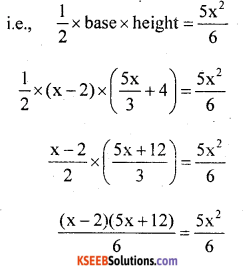x(5x + 12)- 2(5x +12) = 6 x $$\frac{5 x^{2}}{6}$$
5x2 + 12x – 10x – 24 = 5x2
5x2 + 2x – 24 – 5x2 = 0
2x = 24
x = $$\frac{24}{2}$$ = 12
x = 12
$$\frac{5 x}{3}=\frac{5 \times 12}{3}=\frac{60}{3}=20 \mathrm{cm}$$
The base of the triangle is 12 cm and altitude is 20 cm

Question 20.
One of the angles of a triangle is equal to the sum of the other two angles. If the ratio of the other two angles of the triangle is 4 : 5 find the angles of the triangles.
Solution:
The ratio of the two angles is 4 : 5 Let the angles be 4x and 5x. Sum of the two angles = 4x + 5x = 9x. The third angle is equal to sum of the other , two angles i.e., 9x.
Sum of the angles of a traingle
= 180°
9x + 9x = 180
18x = 180
∴ x = $$\frac{180}{8}$$
x = 10°
4x = 4 x 10 = 40°
5x = 5 x 10 = 50°
9x = 9 x 10 = 90° .
∴ The angles of the triangle are 90°, 40° and 50°.error: Content is protected !!